# Python经典练习题（二）

+关注继续查看

## 🍀题目一

rabbits = [1, 1]
# 计算兔子总数的月数
months = 24  # 假设计算24个月的兔子总数，你可以根据需要调整月数
# 计算每个月的兔子总数
for i in range(2, months):
new_rabbits = rabbits[i - 1] + rabbits[i - 2]  # 新生的兔子数等于前两个月的兔子总数之和
rabbits.append(new_rabbits)
# 输出每个月的兔子总数
for i, total in enumerate(rabbits, start=1):
print(f"第{i}个月的兔子总数为：{total}")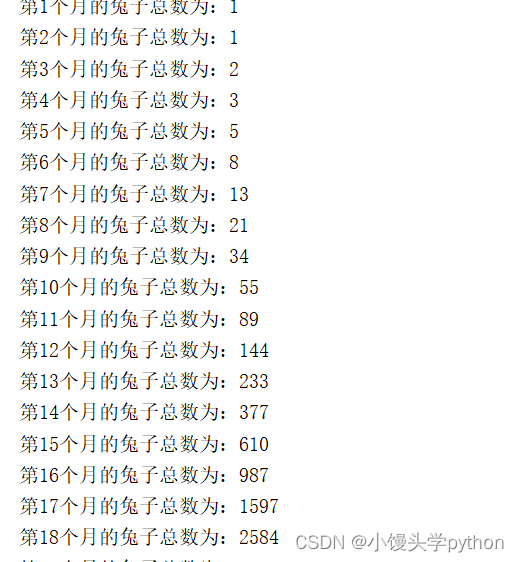## 🍀第二题

# 方法一：使用嵌套循环判断素数
prime_numbers = []  # 存储素数的列表
for num in range(101, 201):  # 遍历101到200之间的所有数字
is_prime = True  # 假设当前数字是素数
# 判断是否为素数
for i in range(2, int(num**0.5) + 1):
if num % i == 0:  # 如果有除1和自身以外的因子
is_prime = False
break
if is_prime:
prime_numbers.append(num)
# 输出所有素数
print("101到200之间的素数有以下", len(prime_numbers), "个：")
print(prime_numbers)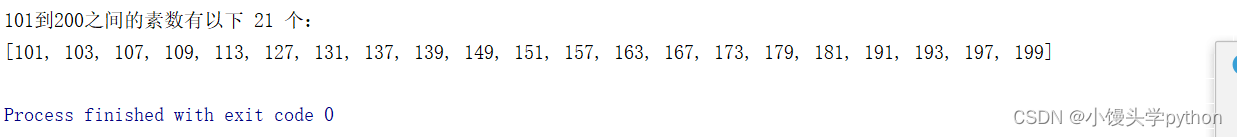# 方法二：使用函数封装判断素数的逻辑
def is_prime(num):
if num < 2:
return False
for i in range(2, int(num**0.5) + 1):
if num % i == 0:
return False
return True
prime_numbers = []  # 存储素数的列表
for num in range(101, 201):  # 遍历101到200之间的所有数字
if is_prime(num):
prime_numbers.append(num)
# 输出所有素数
print("101到200之间的素数有以下", len(prime_numbers), "个：")
print(prime_numbers)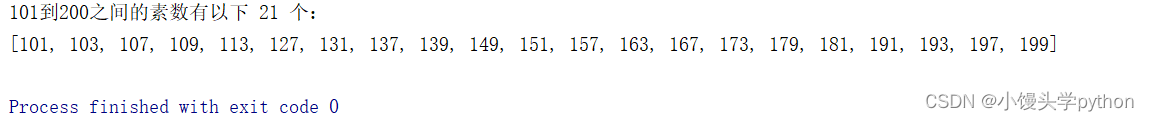## 🍀第三题

for num in range(100, 1000):  # 遍历所有的三位数
# 获取百位、十位和个位上的数字
hundreds = num // 100
tens = (num % 100) // 10
ones = num % 10
# 计算立方和
sum_of_cubes = hundreds ** 3 + tens ** 3 + ones ** 3
# 判断是否为水仙花数
if sum_of_cubes == num:
print(num)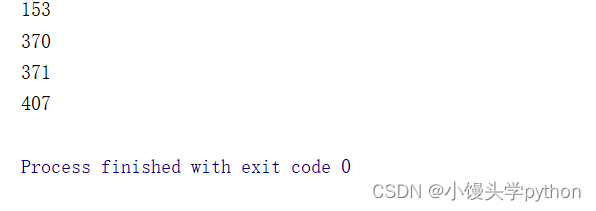## 🍀第四题

def prime_factors(n):
factors = []  # 存储质因数的列表
divisor = 2  # 初始除数为2
while n > 1:
while n % divisor == 0:
factors.append(divisor)  # 将当前除数加入质因数列表
n //= divisor  # 更新n的值，除以当前除数
divisor += 1  # 尝试下一个除数
return factors
# 输入正整数
number = int(input("请输入一个正整数："))
# 计算质因数
factors = prime_factors(number)
# 输出结果
if len(factors) == 0:
print(f"{number}没有质因数，它本身就是一个质数。")
else:
print(f"{number}的质因数分解为：{' * '.join(map(str, factors))}")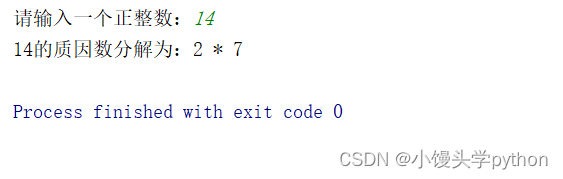## 🍀第五题

def calculate_grade(score):
if 90 <= score <= 100:
return 'A'
elif 80 <= score < 90:
return 'B'
elif 70 <= score < 80:
return 'C'
elif 60 <= score < 70:
return 'D'
elif 0 <= score < 60:
return 'F'
else:
return '无效分数'
# 输入学生分数
score = float(input("请输入学生的分数："))
# 计算并输出等级
print(f"学生的等级为：{grade}")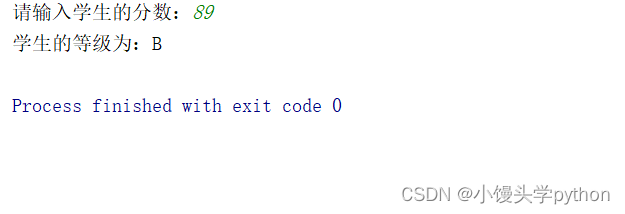|
2月前
|

Python经典练习题（三）
Python经典练习题（三）
49 0
|
2月前
|
Python
Python经典练习题（一）
Python经典练习题（一）
72 0
|
3月前
python--多态和两个练习题
python--多态和两个练习题
24 0
|
5月前
|
Python
Python|PTA练习题
Python|PTA练习题
70 0
|
5月前
|

Python——字符串以及分支语句练习题
Python——字符串以及分支语句练习题
65 0
|
5月前
|

Python——变量以及基础数据类型练习题
Python——变量以及基础数据类型练习题
101 0
|
9月前
|

Python 数学练习题（二）
Python 数学练习题
265 1
|
9月前
|
Python
Python 数学练习题（一）
Python 数学练习题
132 0
|
9月前
|
Python
Python 基础练习题(三)
Python 基础练习题
85 0
|
9月前
|
Python
Python 基础练习题(二)
Python 基础练习题
83 0